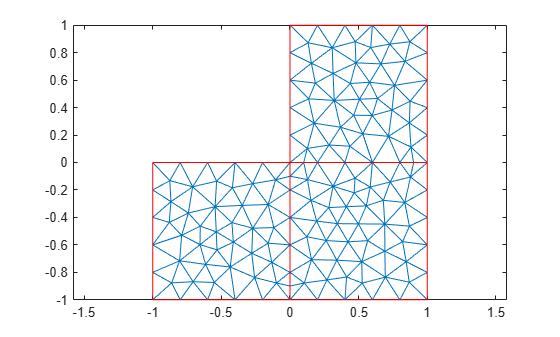initmesh

Create initial 2-D mesh

This page describes the legacy workflow. New features might not be compatible with the legacy workflow. For the corresponding step in the recommended workflow, see generateMesh.

Description

example

[p,e,t] = initmesh(g) generates a triangular mesh for a 2-D geometry. The function uses a Delaunay triangulation algorithm.

example

[p,e,t] = initmesh(g,Name,Value) generates a 2-D mesh using one or more Name,Value pair arguments.

Examples

collapse all

Generate a triangular mesh of the L-shaped membrane.

[p,e,t] = initmesh('lshapeg');

Plot the mesh.

pdemesh(p,e,t)Generate a triangular mesh of the L-shaped membrane with the target maximum mesh edge length of 0.1.

[p,e,t] = initmesh('lshapeg','Hmax',0.1);

Plot the mesh.

pdemesh(p,e,t)Input Arguments

collapse all

Geometry description, specified as a decomposed geometry matrix, a geometry function, or a handle to the geometry function. For details about a decomposed geometry matrix, see decsg. For details about a geometry function, see Parametrized Function for 2-D Geometry Creation.

A geometry function must return the same result for the same input arguments in every function call. Thus, it must not contain functions and expressions designed to return a variety of results, such as random number generators.

Data Types: double | char | string | function_handle

Name-Value Arguments

Specify optional comma-separated pairs of Name,Value arguments. Name is the argument name and Value is the corresponding value. Name must appear inside quotes. You can specify several name and value pair arguments in any order as Name1,Value1,...,NameN,ValueN.

Example: [p,e,t] = initmesh('lshapeg','Hmax',0.1);

Target maximum mesh edge length, specified as the comma-separated pair consisting of 'Hmax' and a positive real number.

Hmax is an approximate upper bound on the mesh edge lengths. initmesh estimates the default value of Hmax from overall dimensions of the geometry.

Small Hmax values let you create finer meshes, but mesh generation can take a very long time in this case. You can interrupt mesh generation by using Ctrl+C. Note that initmesh can take additional time to respond to the interrupt.

Example: [p,e,t] = initmesh(g,'Hmax',0.25);

Data Types: double

Mesh growth rate, specified as the comma-separated pair consisting of 'Hgrad' and a number strictly greater than 1 and less than 2.

Data Types: double

Toggle to preserve bounding box, specified as the comma-separated pair consisting of 'Box' and 'on' or 'off'. By turning on 'Box' you can get a good idea of how the mesh generation algorithm works within the bounding box.

Example: [p,e,t] = initmesh(g,'Box','on');

Data Types: char | string

Toggle to use edge triangulation, specified as the comma-separated pair consisting of 'Init' and 'on' or 'off'. By turning on Init you can see the initial triangulation of the boundaries. For example, use these commands to determine the subdomain number n of the point xy.

[p,e,t] = initmesh(g,'Hmax',Inf,'Init','on');
[uxy,tn,a2,a3] = tri2grid(p,t,zeros(size(p,2)),x,y);
n = t(4,tn);

If the point is outside the geometry, tn is NaN, and the command n = t(4,tn) results in a failure.

Data Types: char | string

Toggle to call jigglemesh after creating the mesh, specified as the comma-separated pair consisting of 'Jiggle' and 'mean', 'minimum''on', or 'off'.

• 'mean' — call jigglemesh with the argument 'Opt' set to 'mean'.

• 'minimum' — call jigglemesh with the argument 'Opt' set to 'minimum'.

• 'on' — call jigglemesh with the argument 'Opt' set to 'off'.

• 'off' — do not call jigglemesh.

Example: [p,e,t] = initmesh(g,'Jiggle','minimum');

Data Types: char | string

Maximum number of iterations for jigglemesh, specified as the comma-separated pair consisting of 'JiggleIter' and a positive integer.

Example: [p,e,t] = initmesh(g,'Jiggle','on','JiggleIter',50);

Data Types: double

Algorithm for generating initial mesh, specified as the comma-separated pair consisting of 'MesherVersion' and either 'R2013a' or 'preR2013a'. The 'R2013a' algorithm runs faster, and can triangulate more geometries than the 'preR2013a' algorithm. Both algorithms use Delaunay triangulation.

Data Types: char | string

Output Arguments

collapse all

Mesh points, returned as a 2-by-Np matrix. Np is the number of points (nodes) in the mesh. Column k of p consists of the x-coordinate of point k in p(1,k) and the y-coordinate of point k in p(2,k). For details, see Mesh Data as [p,e,t] Triples.

Mesh edges, returned as a 7-by-Ne matrix, where Ne is the number of boundary edges in the mesh. An edge is a pair of points in p containing a boundary between subdomains, or containing an outer boundary. For details, see Mesh Data as [p,e,t] Triples.

Mesh elements, returned as a 4-by-Nt matrix. Nt is the number of triangles in the mesh.

The t(i,k), with i ranging from 1 through end - 1, contain indices to the corner points of element k. For details, see Mesh Data as [p,e,t] Triples. The last row, t(end,k), contains the subdomain number of the element.

 George, P. L. Automatic Mesh Generation — Application to Finite Element Methods. Wiley, 1991.

Partial Differential Equation Toolbox DocumentationTry MATLAB, Simulink, and Other Products

Get trial now